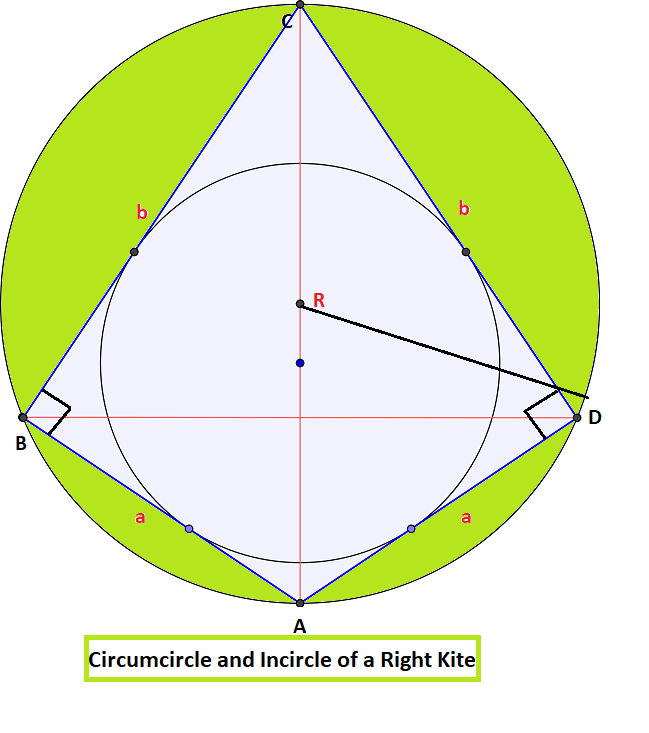# Area of Circumcircle and Incircle of a Right Kite

• Difficulty Level : Expert
• Last Updated : 20 Jul, 2021

Given two positive integers A and B representing the sides of the right kite, the task is to find the area of the circumcircle and incircle of a right kite.

A right kite is a kite that can be inscribed in a circle with two opposite angles are at right angles. The line of symmetry of the kite is also the diameter of the circumcircle of the kite. It divides the kite into two congruent right-angled triangles having sides as A and B of a right kite.

Attention reader! Don’t stop learning now. Get hold of all the important DSA concepts with the DSA Self Paced Course at a student-friendly price and become industry ready.  To complete your preparation from learning a language to DS Algo and many more,  please refer Complete Interview Preparation Course.

In case you wish to attend live classes with experts, please refer DSA Live Classes for Working Professionals and Competitive Programming Live for Students.Examples:

Input: A = 3, B = 4
Output: Area of circumcircle of Right Kite is 19.625, Area of incircle of Right Kite is 3.14

Input: A = 10, B = 5
Output: Area of circumcircle of Right Kite is 98.125, Area of incircle of Right Kite is 28.26

Approach: There are some observations to solve this problem. Follow the steps below to solve this problem:

• Here, a = AB = AD and b = BC = CD
• In the kite ABCD with opposite angles B and D as 90°, thus the opposite angles can be calculated as tan (A/2) = b/a and tan(C/2) = a/b
• Let p as the length of diagonal AC and q as the length of diagonal BD.
• Diagonal AC can be easily calculated using the Pythagoras Theorem. Hence p = (a2 + b2)½
• Since the diagonal is equal to the diameter of the circumcircle of the kite, the radius of the circumcircle is calculated as R = (a2 + b2)½/2
• Thus, the area of the circumcircle will be pi * R* R
• Also, all kites are tangential quadrilaterals, therefore the radius of the incircle can be calculated by r = Area of kite/Semiperimeter of the kite i.e r = a*b/(a+b).
• Thus, the area of the incircle will be pi*r*r.

Below is the implementation of the above approach:

## C++

 `// C++ program for the above approach` `#include ``using` `namespace` `std;``#define pi 3.14` `// Function to calculate the area of``// circumcircle of right kite``double` `AreaOfCircumcircle(``int` `a, ``int` `b)``{``    ``// Find the radius``    ``double` `radius = ``sqrt``(a * a + b * b)``                    ``/ 2;``    ``return` `pi * radius * radius;``}` `// Function to calculate the area of``// incircle of right kite``double` `AreaOfIncircle(``int` `a, ``int` `b)``{``    ``// Find the radius``    ``double` `radius = (a * b) / (a + b);` `    ``return` `pi * radius * radius;``}` `// Driver Code``int` `main()``{``    ``// Given Input``    ``int` `a, b;``    ``a = 10;``    ``b = 5;` `    ``// Function Call``    ``double` `circumarea = AreaOfCircumcircle(``        ``a, b);``    ``cout << ``"Area of circumcircle of Right Kite is"``         ``<< ``" "` `<< circumarea << endl;` `    ``// Function Call``    ``double` `inarea = AreaOfIncircle(``        ``a, b);``    ``cout << ``"Area of incircle of Right Kite is"``         ``<< ``" "` `<< inarea << endl;` `    ``return` `0;``}`

## Java

 `// Java program for the above approach``public` `class` `GFG {``    ``static` `double` `pi = ``3.14``;` `    ``// Function to calculate the area of``    ``// circumcircle of right kite``    ``static` `double` `AreaOfCircumcircle(``int` `a, ``int` `b)``    ``{``        ``// Find the radius``        ``double` `radius = Math.sqrt(a * a + b * b) / ``2``;``        ``return` `pi * radius * radius;``    ``}` `    ``// Function to calculate the area of``    ``// incircle of right kite``    ``static` `double` `AreaOfIncircle(``int` `a, ``int` `b)``    ``{``        ``// Find the radius``        ``double` `radius = (a * b) / (a + b);` `        ``return` `pi * radius * radius;``    ``}``  ` `    ``// Driver code``    ``public` `static` `void` `main(String[] args)``    ``{``      ` `        ``// Given Input``        ``int` `a, b;``        ``a = ``10``;``        ``b = ``5``;` `        ``// Function Call``        ``double` `circumarea = AreaOfCircumcircle(a, b);``        ``System.out.printf(``            ``"Area of circumcircle of Right Kite is %.3f\n"``,``            ``circumarea);` `        ``// Function Call``        ``double` `inarea = AreaOfIncircle(a, b);``        ``System.out.printf(``            ``"Area of incircle of Right Kite is %.2f\n"``,``            ``inarea);``    ``}``}` `// This code is contributed by abhinavjain194`

## Python3

 `# Python program for the above approach``# Function to calculate the area of``# circumcircle of right kite``import` `math``pi ``=` `3.14` `def` `AreaOfCircumcircle(a, b):` `    ``# Find the radius``    ``radius ``=` `math.sqrt(a ``*` `a ``+` `b ``*` `b)``/` `2``    ``return` `pi ``*` `radius ``*` `radius`  `# Function to calculate the area of``# incircle of right kite``def` `AreaOfIncircle( a,  b):` `    ``# Find the radius``    ``radius ``=` `(a ``*` `b) ``/``/` `(a ``+` `b)``    ``return` `pi ``*` `(radius``*``*``2``)`  `# Driver Code``# Given Input``a ``=` `10``b ``=` `5` `# Function Call``circumarea ``=` `AreaOfCircumcircle(a, b)``print``(``"Area of circumcircle of Right Kite is"` `,``" "` `, ``format``(circumarea,``".3f"``))` `# Function Call``inarea ``=` `AreaOfIncircle(a, b)``print``(``"Area of incircle of Right Kite is"` `,``" "` `, ``format``(inarea,``".2f"``))` `# this code is contributed by shivanisinghss2110`

## C#

 `// C# program for the above approach``using` `System;` `class` `GFG{``    ` `static` `double` `pi = 3.14;` `// Function to calculate the area of``// circumcircle of right kite``static` `double` `AreaOfCircumcircle(``int` `a, ``int` `b)``{``    ` `    ``// Find the radius``    ``double` `radius = Math.Sqrt(a * a + b * b) / 2;``    ``return` `pi * radius * radius;``}` `// Function to calculate the area of``// incircle of right kite``static` `double` `AreaOfIncircle(``int` `a, ``int` `b)``{``    ` `    ``// Find the radius``    ``double` `radius = (a * b) / (a + b);` `    ``return` `pi * radius * radius;``}` `// Driver code``public` `static` `void` `Main()``{``    ` `    ``// Given Input``    ``int` `a, b;``    ``a = 10;``    ``b = 5;` `    ``// Function Call``    ``double` `circumarea = AreaOfCircumcircle(a, b);``    ``Console.WriteLine(``        ``"Area of circumcircle of Right Kite is "` `+``        ``circumarea);` `    ``// Function Call``    ``double` `inarea = AreaOfIncircle(a, b);``    ``Console.WriteLine(``        ``"Area of incircle of Right Kite is "` `+ inarea);``}``}` `// This code is contributed by subhammahato348`

## Javascript

 ``
Output
```Area of circumcircle of Right Kite is 98.125
Area of incircle of Right Kite is 28.26```

Time Complexity: O(1)
Auxiliary Space: O(1)

My Personal Notes arrow_drop_up